C++ Lesson 32:  Linked Lists

INTRODUCTION:               In this lesson you begin to study dynamic data structures linked together with pointers.  We will start out with a complete example program, then you will complete a lab exercise to practice what you have learned.

The key topics for this lesson are:

A.  Declarations for a Linked List

B.   Building a Linked List - function insert

C.  Using struct Constructors

D.  Traversing a Linked List - function printList

E.   Pitfalls of Linked Data Structures

F.   Value vs. Reference Parameters with Pointers

VOCABULARY:                   NODE           LINKED LIST       TRAVERSE

DISCUSSION:                       A.  Declarations for a Linked List

1.   A single pointer variable by itself is rather useless.  However a self-referencing structure will allow us to create a linked data structure.

struct listNode

{

int  data;

listNode  *next;

};

2.   The declaration seems circular and in some ways it is, but the compiler will allow such definitions.  A listNode will have two data members, an integer and a pointer.  The pointer variable next will point to the next listNode in a linked list.

3.   We can now declare pointer variables which point to a listNode.

listNode  *list;

The pointer variable list will eventually hold an address of where a listNode is stored in memory.

4.   The following short program using listNode will illustrate the syntax of accessing the data members of a listNode via a pointer.

Program 32-1

#include <iostream.h>

struct  listNode

{

int  data;

listNode  *next;

};

main()

{

listNode  *list;

list = new  listNode;

(*list).data = 5;

(*list).next = NULL;

}

This results in this type of memory allocation and data storage.The diagonal line in the next field is a pictorial representation of a NULL (0) value.

5.   The statement  (*list).data = 5;   needs some explanation.  The variable list is a pointer variable, the expression (*list) dereferences list - we are about to work with the memory location stored in list.  The dot notation (.data) means that we wish to work with the value data inside of the location pointed to by list.  The parentheses are needed because the dot operator has priority over the dereferencing operator.  If you leave out the parentheses, the expression *list.data, would attempt to dereference list.data, which is not a pointer but simply an integer value.

6.   C++ provides a cleaner alternative to the above syntax.  The member selection operator (->) will accomplish the same result of using the indirection operator (*) in conjunction with the dot operator.  These statements are equivalent:

list->data = 5;       is the same as    (*list).data = 5;

The member selection operator will be used throughout the rest of the curriculum guide.

7.   The result of program 32-1 is a pointer (list) which points to a single structure.  The next field inside of the node does not point anywhere.  If we use the next field to link nodes together, we will have a data structure called a linked list.  The next section will walk you through the construction of a simple linked list.

8.   C++ provides a typedef statement which enables us to make an alias of a data type.  This will allow us to write more compact variable declarations and parameter lists.

typedef  listNode  *listPtr;

The identifier listPtr is an alias for listNode *.  In Program 32-1, the statement,

listNode  *list;     could be replaced with     listPtr  list;

The variable list is of type listPtr, which really is an alias type for listNode*.

B.   Building a Linked List - function insert

 See Handout H.A.32.1, listDemo.cpp. 1.   An example program, which builds a linked list with 5 nodes, is provided as Handout H.A.32.1, listDemo.cpp.  Section B of the student outline will refer to the left-hand program on Handout H.A.32.1, (the version without a struct constructor).  The source of the data is the for loop inside function createList.

2.   The appearance of the final version of the linked list is: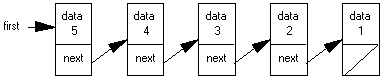3.   The insert function is the key to understanding this first program on linked lists.  The function receives root as a reference parameter.  This makes root an alias pointer for variable first in function main.  The new integer, value, is to be placed at the head of the list.  In the diagrams, the head or front of the list is on the left.

4.   When the first call of insert is made, root is NULL and value equals 1.  The following two lines results in the allocation of memory and the assignment of 1 to the data field of newNode.

newNode = new  listNode;

newNode->data = value;

These two steps can be described as packaging the node.5.   The remaining two steps rehook pointers to establish a linked list of one node.

newNode->next = root;

This transfers NULL from root to newNode->next.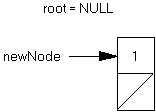The next line updates the value of root from NULL to the address stored in newNode.

root = newNode;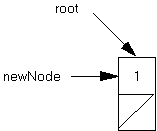6.   When the function ends, the local identifier, newNode, is destroyed.  Because root was passed as a reference parameter, the pointer first in function main now points to a new memory location instead of the NULL value it had before the first call of function insert.7.   The second call of function insert receives root pointing to the list above and the integer value 2.  A newNode is allocated, the 2 is placed inside the node, and the first pointer hookup produces this diagram

newNode = new  listNode;

newNode->data = value;

newNode->next = root;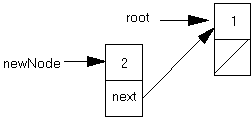then, root = newNode;8.   The insert function terminates and the pointer variable first (in function main) points to the newly added node in the list, which in turn points to the next node.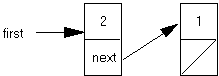C.  Using struct Constructors

 See Handout H.A.32.1, listDemo.cpp. 1.   C++ provides for struct constructors which will shorten the code in dynamic data structure programs.  Handout H.A.32.1, listDemo.cpp,  has a parallel program for listDemo.cpp using a struct constructor.

2.   The struct constructor interface is written inside of the struct declaration area.  The actual code for the constructor is placed outside of the struct declaration, hence the need for the scope resolution operator.

struct listNode

{

int  data;

listNode  *next;

listNode(int, listNode *);

};

listNode::listNode (int tempData, listNode *tempNext)

: data(tempData), next(tempNext)

{

// all values initialized in initializer list

}

The name of the struct constructor must match the identifier which names the struct.  The parameters tempData and tempNext will be assigned to the data fields of a newly constructed node.  Notice the use of an initializer list instead of direct assignment statements.

3.   The insert function is no longer needed in this version and the createList function uses the struct constructor.

void createList (nodePtr &root)

{

root = NULL;

for (int k=1; k<=5; k++)

root = new listNode (k, root);

}

4.   The statement  listNode (k, root)  invokes the struct constructor.  The line of code

root = new listNode (k, root);

is broken down as follows:

a.   The new command allocates enough memory to store a listNode.

b.   The new listNode will be assigned the values of k and root.

c.   The address of this newly constructed listNode is assigned to root.

d.   It is important to understand the old and new values of root: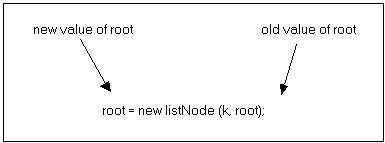5.   When k = 1, root = NULL.  A new node is constructed with the  values 1 and NULL.  This new node is pointed to by root.  In a sense, the struct constructor provides a new node between the variable root and the node which root used to point to.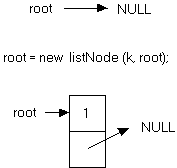before the call of the constructor   call the constructor, root is passed as a NULL value   root is changed, points to the newly constructed node

6.   When k = 2, root is already pointing to the first node of the linked list.  When the constructor is called, the new node is constructed and placed between root and the node root used to point to.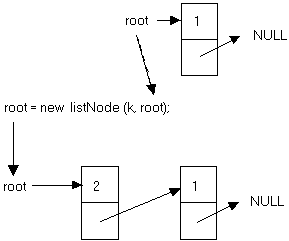The value of root passed to the struct constructor is used to initialize the next field of the new node.

D.  Traversing a Linked List - function printList

1.   To traverse a list means to start at one end and visit all the nodes, solving a problem along the way.  In the case of function printList, the task is to print the data field from each node.

void printList (nodePtr temp)

{

while (temp != NULL)

{

cout << temp->data << "   ";

temp = temp->next;

}

cout << endl;

}

a.   Because temp is passed as a value parameter, we can use it to traverse the list.  The pointer temp will contain NULL when we are done.  Since it is not an alias, we protect the value of first in function main.

b.   Until temp equals NULL, the while loop will do two steps at each node, print the data field, then advance the temp pointer.

c.   The statement, temp = temp->next, is a very important one as this is how we move a pointer to the next node.  The following diagrams with addresses illustrate this key statement.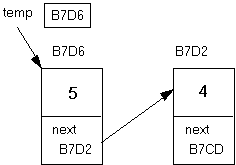The pointer temp is storing (pointing at) address B7D6.  The assignment statement, temp = temp->next, assigns the address B7D2 (the value of temp->next) to temp.  In other words, whatever node temp is pointing at, it now stores the address inside of that node's next field.  The diagram now changes to:d.  This loop will continue until temp equals NULL.

2.   Another common list traversal problem is counting the number of nodes in the list.  The lab exercise will ask you to solve this problem.

E.   Pitfalls of Linked Data Structures

1.   A linked list must end with a NULL value.  Without such a marker at the end of the list, a routine cannot “see” the end of the data structure.  This assignment of a NULL value at the end of the list is often taken care of when a new node is packaged or through the use of a struct constructor.

2.   The program must not inspect a NULL location.  If a pointer equals NULL, there is no address to inspect and therefore attempting to access a field of a non-existent node will cause a program crash.  If first == NULL, a statement like first->data will result in a run-time error because NULL is not a memory location.

3.   The function which adds a node to a linked data structure must receive a pointer to the data structure as a reference parameter.  When the first node is added to an empty data structure, the variable which points to the list will change from NULL to a memory address.

4.   Every time (EVERY TIME!) a new node is inserted into a dynamic data structure, memory must be allocated using the new command.  The new command will be followed by explicit assignments to the data members of a node or the use of a struct constructor.

5.   However, new must not be used any other time.  Here is a common error when programming with pointers.

void printList (nodePtr temp)

{

nodePtr  local;

local = new  listNode;

local = temp;

while (local != NULL)

{

cout << local->data << "   ";

local = local->next;

}

cout << endl;

}

When local is declared as a local pointer variable, it is ready to store an address.  The assignment of a new memory address to local is not necessary.  The effect of the code above is to allocate memory and store that address in local, which then receives the address of temp, effectively losing track of the allocated memory.  We have wasted memory.

F.   Value vs. Reference Parameters with Pointers

1.   The function printList uses a value parameter which makes temp a local variable only.  This protects the value of pointer variable first in function main from any changes.  However, this does not protect the contents in the nodes of the list from changes.

2.   When a pointer is passed as a value parameter, the contents of the memory location it points to can be permanently changed.  Assume first points to a list of five nodes and the following function call is made.

main()

{

... initialize list pointed to by first

changeFirstNode (first);

return 0;

}

void changeFirstNode (listPtr temp)

//  changes data field of the first node to zero

{

temp->data = 0;

}

When this function has completed and program control returns to function main, the location first points to has not changed. However the contents of the memory location it points to have been changed.

3.   A misconception exists that a pointer passed as a value parameter protects the data structure from any permanent global changes.  Because pointers allow you to directly inspect memory locations, changes can be made to a list.

void  zeroList (listPtr  temp)

//  changes all data fields to zero

{

while (temp != NULL)

{

temp->data = 0;

temp = temp->next;

}

}

4.   Lastly, a pointer passed as a reference parameter can have its contents changed as well as the contents of the location it points to.  Predict the effect of this function:

void  zeroList (listPtr  &temp)

//  changes all data fields to zero and ???

{

while (temp != NULL)

{

temp->data = 0;

temp = temp->next;

}

}

SUMMARY/REVIEW:         You have just been taught your first dynamic data structure, a linked list.  The concept of indirection makes learning pointers a bit more difficult, but careful reading and lots of diagrams will help.  Following a working program is a good start; but only by writing code will you develop proficiency with pointers.  The lab exercises for the next few lessons will test your understanding of pointers.

ASSIGNMENT:                    Lab Exercise L.A.32.1, List1

Answer to the last section in the student outline:      All the nodes in the linked list now have the value 0.  More importantly, the original pointer passed as a parameter to this function now points to NULL.  This is not good.